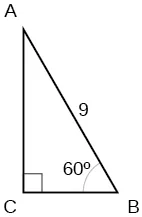Algebra and Trigonometry

# Practice Test

Algebra and TrigonometryPractice Test

### Practice Test

1.

Convert $5π 6 5π 6$ radians to degrees.

2.

Convert $−620° −620°$ to radians.

3.

Find the length of a circular arc with a radius 12 centimeters subtended by the central angle of $30°. 30°.$

4.

Find the area of the sector with radius of 8 feet and an angle of $5π 4 5π 4$ radians.

5.

Find the angle between $0° 0°$ and $360° 360°$ that is coterminal with $375°. 375°.$

6.

Find the angle between 0 and $2π 2π$ in radians that is coterminal with $− 4π 7 . − 4π 7 .$

7.

Draw the angle $315° 315°$ in standard position on the Cartesian plane.

8.

Draw the angle $− π 6 − π 6$ in standard position on the Cartesian plane.

9.

A carnival has a Ferris wheel with a diameter of 80 feet. The time for the Ferris wheel to make one revolution is 75 seconds. What is the linear speed in feet per second of a point on the Ferris wheel? What is the angular speed in radians per second?

10.

Find the missing sides of the triangle $ABC:sinB= 3 4 ,c=12. ABC:sinB= 3 4 ,c=12.$

11.

Find the missing sides of the triangle.12.

The angle of elevation to the top of a building in Chicago is found to be 9 degrees from the ground at a distance of 2000 feet from the base of the building. Using this information, find the height of the building.

13.

Find the exact value of $sin π 6 . sin π 6 .$

14.

Compute sine of $240°. 240°.$

15.

State the domain of the sine and cosine functions.

16.

State the range of the sine and cosine functions.

17.

Find the exact value of $cot π 4 . cot π 4 .$

18.

Find the exact value of $tan π 3 . tan π 3 .$

19.

Use reference angles to evaluate $csc 7π 4 . csc 7π 4 .$

20.

Use reference angles to evaluate $tan210°. tan210°.$

21.

If $csct=0.68, csct=0.68,$ what is the $csc(−t)? csc(−t)?$

22.

If $cost= 3 2 , cost= 3 2 ,$ find $cos(t−2π). cos(t−2π).$

23.

Find the missing angle: $cos( π 6 )=sin( ___ ) cos( π 6 )=sin( ___ )$

Order a print copy

As an Amazon Associate we earn from qualifying purchases.